# obliqueslice

Extract oblique slice from 3-D volumetric data

Since R2020a

## Syntax

``B = obliqueslice(V,point,normal)``
``B = obliqueslice(V,point,normal,Name=Value)``
``[B,x,y,z] = obliqueslice(___)``

## Description

example

````B = obliqueslice(V,point,normal)` extracts a 2-D oblique slice from a 3-D volumetric data `V`. The slice is extracted with reference to a given point on the volume and a normal vector. The slicing plane is perpendicular to the normal vector and passes through the specified point.For information about how the slice is extracted with respect to the given point and the normal, see Oblique Slicing.```

example

````B = obliqueslice(V,point,normal,Name=Value)` specifies options using one or more name-value arguments.```

example

````[B,x,y,z] = obliqueslice(___)` also returns the 3-D Cartesian coordinates of the extracted slice in the input volume. For information about how the intensity values at these 3-D coordinates are mapped to 2-D plane, see Mapping Values from 3-D Coordinate Space to Image Plane.```

## Examples

collapse all

Load a 3-D volumetric data set into the workspace.

`load mri`

Remove singleton dimensions by using the `squeeze` function.

`V = squeeze(D);`

Display horizontal slices of the data by using the `montage` function.

`montage(V,map,'Size',[3 9]);`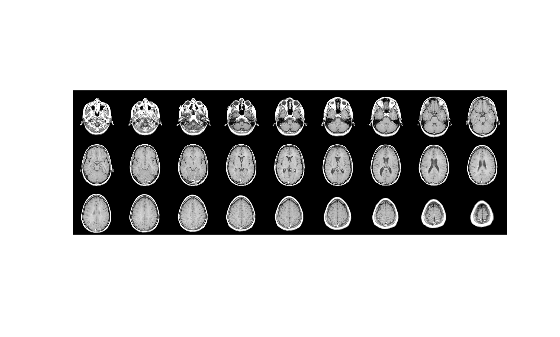Specify a point in the volume for the slice to pass through.

`point = [73 50 15.5];`

Specify a normal vector in 3-D coordinate space.

`normal = [0 15 20];`

Extract a slice from the volumetric data. The slice is perpendicular to the normal vector and passes through the specified point.

`[B,x,y,z] = obliqueslice(V,point,normal);`

Display the extracted slice in the 3-D coordinate space.

```figure surf(x,y,z,B,'EdgeColor','None','HandleVisibility','off'); grid on view([-38 12]) colormap(gray) xlabel('x-axis') ylabel('y-axis'); zlabel('z-axis'); title('Slice in 3-D Coordinate Space')```

Plot the point and the normal vector.

```hold on plot3(point(1),point(2),point(3),'or','MarkerFaceColor','r'); plot3(point(1)+[0 normal(1)],point(2)+[0 normal(2)],point(3)+[0 normal(3)], ... '-b','MarkerFaceColor','b'); hold off legend('Point in the volume','Normal vector')```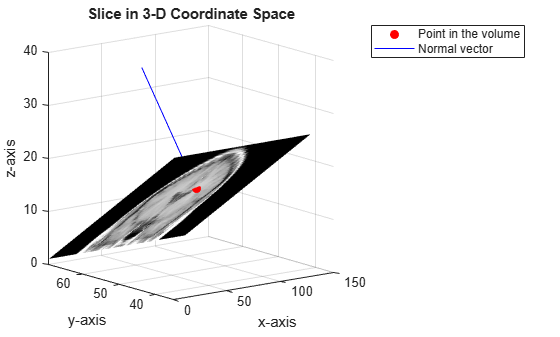Display the extracted slice in the image plane.

```figure imshow(B,[]) title('Slice in Image Plane')```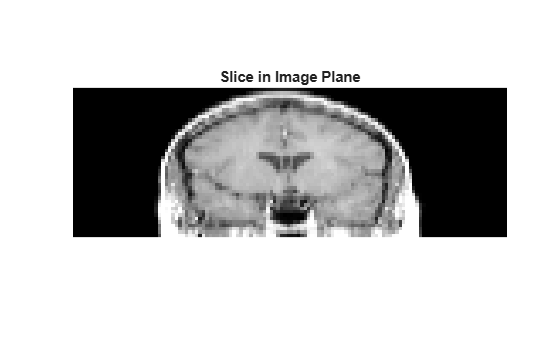Load a 3-D volumetric data set into the workspace.

```s = load(fullfile(toolboxdir('images'),'imdata','BrainMRILabeled','images','vol_001.mat')); V = s.vol;```

Display horizontal slices of the data by using the `montage` function.

`montage(V,'Indices',12:118,'Size',[8 12],'DisplayRange',[]);`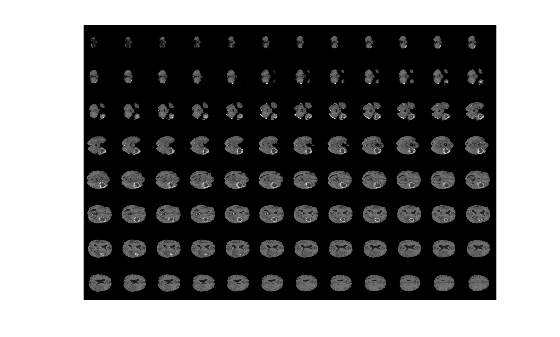Specify the normal vector to a plane in 3-D coordinate space.

`normal = [20 0 10];`

Extract multiple slices along the direction of the normal vector using a `for` loop. In each iteration:

• Specify a point that the slice has to pass through.

• Extract the slice, specifying the output size to `'Full'` and the fill value for padding pixels as 255. The extracted slices are perpendicular to the normal vector and pass through the specified point.

• Display the extracted slices.

```sliceIdx = 10:5:180; figure for s = 1:length(sliceIdx) pt = [sliceIdx(s) 150 80]; [B,x,y,z] = obliqueslice(V,pt,normal,'OutputSize','Full','FillValues',255); Bslices(:,:,s) = B; % Display the slice in 3-D coordinate space subplot('Position',[0.11 0.36 0.38 0.5]) surf(x,y,z,B,'EdgeColor','None','HandleVisibility','off'); grid on view([-24 12]) colormap(gray) xlabel('x-axis') ylabel('y-axis'); zlabel('z-axis'); zlim([0 155]); ylim([0 250]); xlim([0 250]); title('Slice in 3-D Coordinate Space') % Plot the point and the normal vector. hold on plot3(pt(1),pt(2),pt(3),'or','MarkerFaceColor','r') plot3( ... pt(1)+[-normal(1) normal(1)], ... pt(2)+[-normal(2) normal(2)], ... pt(3)+[-normal(3) normal(3)], ... '-b','MarkerFaceColor','b') legend('Point in the volume','Normal vector','Position',[0.1 0.12 0.3 0.08]) hold off % Display the extracted slice. subplot('Position',[0.6 0.37 0.34 0.49]) imshow(B,[]) title('Slice in Image Plane') pause(0.5); end ```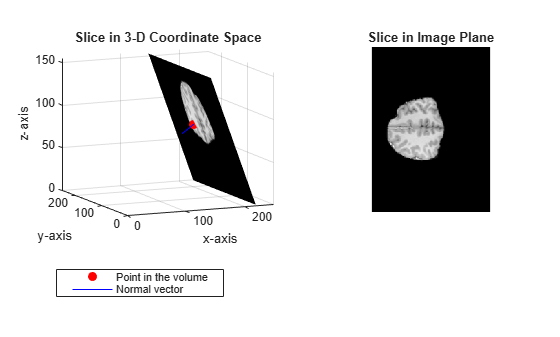Display the extracted image slices by using the `montage` function.

```figure montage(Bslices,'Size',[5 7],'DisplayRange',[]);```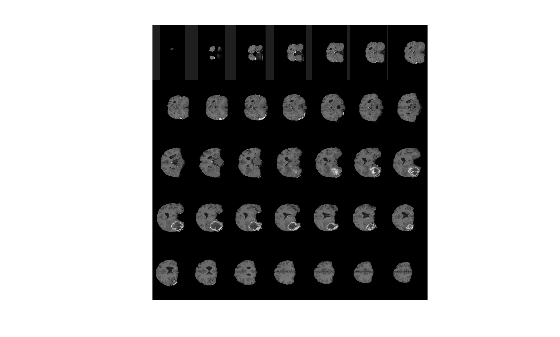## Input Arguments

collapse all

Input volume, specified as a 3-D numeric array or 3-D categorical array.

Data Types: `single` | `double` | `int8` | `int16` | `int32` | `uint8` | `uint16` | `uint32` | `logical` | `categorical`

Point in the volume, specified as a 3-element row vector of the form [px py pz].

Data Types: `single` | `double` | `int8` | `int16` | `int32` | `int64` | `uint8` | `uint16` | `uint32` | `uint64`

Normal vector, specified as a 3-element row vector of form [`a` `b` `c`]. The normal vector is a vector that is perpendicular to a surface or plane.

To extract an orthogonal slice, you can set the normal vector to one of these values:

• `[1 0 0]` — Extract slice in the yz-plane.

• `[0 1 0]` — Extract slice in the xz-plane.

• `[0 0 1]` — Extract slice in the xy-plane.

Data Types: `single` | `double` | `int8` | `int16` | `int32` | `int64` | `uint8` | `uint16` | `uint32` | `uint64`

### Name-Value Arguments

Specify optional pairs of arguments as `Name1=Value1,...,NameN=ValueN`, where `Name` is the argument name and `Value` is the corresponding value. Name-value arguments must appear after other arguments, but the order of the pairs does not matter.

Example: `obliqueslice(V,point,normal,OutputSize="Full")`

Before R2021a, use commas to separate each name and value, and enclose `Name` in quotes.

Example: `obliqueslice(V,point,normal,"OutputSize","Full")`

Interpolation method, specified as one of these values:

• `"linear"` — linear interpolation

• `"nearest"` — nearest neighbor interpolation

If `V` is numeric, the interpolation method defaults to `"linear"` but can also be specified as `"nearest"`. If `V` is categorical, then the interpolation method must be `"nearest"`.

Data Types: `char` | `string`

Size of output image, specified as one of these values:

• `"limit"` — The size of the output image is the actual size of the 2-D slice with respect to the dimensions of input volume. If the extracted slice region is not square or rectangular, the function automatically pads the extracted slice region with extra pixels to yield a square or rectangular image.

• `"full"` — The size of the output image may not be equal to the actual size of the 2-D slice. The size of the output image is set to the maximal slice size that can be obtained from the input volume with respect to the normal vector `normal`. To resize the image, the border of the extracted 2-D slice is padded with extra rows and columns.

The fill value for the padded pixels is `0` by default. You can use the `FillValues` name-value argument to change the value.

Data Types: `char` | `string`

Fill value for padded pixels, specified as a numeric scalar, character vector, or `missing`.

When `V` is a numeric array, specify

• `0` for zero padding.

• numeric scalar for constant padding.

When `V` is a categorical array, specify

• character vector that denotes a category in the input data. To know the categories, use the `categories` function.

• `missing`, if the category in input data is equal to `<undefined>`.

Data Types: `single` | `double` | `int8` | `int16` | `int32` | `int64` | `uint8` | `uint16` | `uint32` | `uint64` | `char`

## Output Arguments

collapse all

Output 2-D slice, returned as a numeric or categorical matrix. The data type of the output slice is same as the data type of the input volume.

Data Types: `single` | `double` | `int8` | `int16` | `int32` | `uint8` | `uint16` | `uint32` | `logical` | `categorical`

x-coordinates of the output slice in the 3-D volume, returned as a numeric matrix of the size same as the output slice, `B`.

Data Types: `single`

y-coordinates of the output slice in the 3-D volume, returned as a numeric matrix of the size same as the output slice, `B`.

Data Types: `single`

z-coordinates of the output slice in the 3-D volume, returned as a numeric matrix of the size same as the output slice, `B`.

Data Types: `single`

collapse all

### Oblique Slicing

Given a point (px, py, pz) and the normal vector (a, b, c), the function solves the plane equation a(x-px)+b(y-py)+c(z-pz) = 0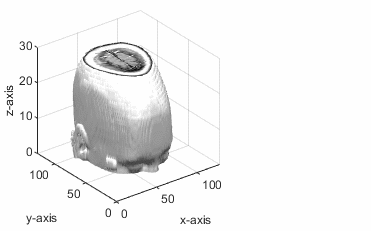The point (px, py, pz) lies in the volumetric data. The slicing plane is perpendicular to the normal vector and passes through the given point.

### Mapping Values from 3-D Coordinate Space to Image Plane

The order in which the coordinates of the extracted slice in 3-D space is mapped to a 2-D plane depends on its inclination angle with respect to the horizontal and vertical planes.

The `obliqueslice` function returns the output matrices `x`, `y`, and `z` that contain the x, y, z coordinates of the points in 3-D coordinate space that form the image slice. The `obliqueslice` function interpolates the intensity values at these points and maps it to the 2-D plane. The first value in the output matrices x(1,1), y(1,1), z(1,1) specify 3-D coordinate of a point that maps as the upper-left pixel, (1, 1) in the image plane. Starting from this point, the 3-D coordinates that constitute the image slice along with the associated intensity values are read in left-to-right, top-to-bottom scan order. These intensity values fill the 2-D image plane in the same left-to-right, top-to-bottom scan order.

Suppose V is the input volumetric data and B is the output 2-D image, then B(i,j) = V(a,b,c).

Where, a = x(i,j), b = y(i,j), and c = z(i,j).## Version History

Introduced in R2020a

expand all